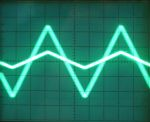﻿ what is triangular wave generator?Back

## what is triangular wave generator?Triangular wave  generator

Introduction

It is designed to operate under 5 Volts, but different voltages can be applied as well, taking in account the maximum operation voltage of the OP-AMPS. The two op-amps currently used are the known 741 chips. Different OP-Amps can be used as well, and also dual chips for simplicity. The right OP-Amp will operate as an integrator and the left as a comparator. When power is given to the circuit, the comparator drives it's output HIGH. This signal is driven to the integrator through the resistor R. The capacitor C then starts to charge gradually with RC time constant. While the capacitor is charging, the output of the integrator is also taken to it's low state with the same rate. When the positive input of the comparator, through the voltage divider that the 47K and 100K resistors perform, is driven low enough, then it changes state, and the integrator starts operating vice-versa.It is easily understood that the frequency of oscillation will only have to do with the RC standard. That is true. A half cycle period is exactly the result of the R x C. A full cycle is twice this amount. Therefore, the frequency is: In our test circuit, the R resistor is 22K and the C capacitor is 100nF. The oscillation frequency would be: And that would make 227,27Hz approximately. In real life, the frequency measured was about 218Hz. This is a rather small (tiny) difference between the theoretical and the practical value, considering that the resistors have 5% accuracy and so does the capacitor as well.

 FOSC = 1 2 x R x    C

If you watch the output signal in a oscilloscope, then you will notice that the triangle waveform is above of the zero voltage. The offset is caused by DC voltage. In order to eliminate this voltage shift, you should add a capacitor in series to the circuit. The value of the capacitor should be chosen according to the oscillation frequency of the circuit. For low frequencies, 1-100 Hz, a 4.7uF to 10uF would work just fine. Above you should consider using smaller capacitors. A wrong capacitor selection would cause signal distortion and sometimes will add significant resistance to the output. The following circuit demonstrates the previous circuit with a series capacitor.

Working of triangular  wave generator

• Comparator compares the voltage at a point ‘P’ continuously with respect to the voltage at the inverting input, which is at ground potential.
• When the voltage at P goes slightly below zero, the output of comparator will switches to negative saturation.
• Consider the output of comparator is +Vsat, since this voltage is the input of integrator, then its output will be negative going ramp.
• Thus one end of the potential divider R1 R2 is at +Vsat and other end is at negative going ramp. When the negative going ramp attains a value say –Vramp the effective voltage at P becomes slightly less than 0V. This switches output of comparator to –Vsat.
• During this time integrator output will be positive going ramp. When the value of positive going ramp attains +Vramp, voltage at ‘P’ becomes slightly greater than 0V, there by switching comparator output to +Vsat.
• This cycle repeats and generates a triangular waveform
• Triangular waveform can also be generated by integrating square wave from an astable multivibrator.